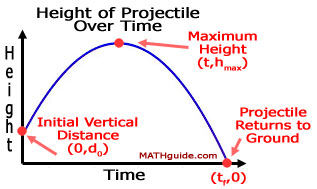Flight of a Projectile
View the Lesson | MATHguide homepage Updated August 3rd, 2021

Waiting for your responses.Hint: Use a graphing calculator to help solve this problem.

 Given: A projectile is launched upward at a velocity of 360 ft/sec on Earth. The projectile starts at an initial vertical distance of 40 feet. Find: a) Write the function that describes the height of the projectile with respect to time.    h(t) = t2 + t + b) At what time does the projectile reach the maximum height?[Round your answer to the nearest tenth.]     seconds c) Determine the maximum height of the projectile.[Round the answer to the nearest foot.]     feet d) At what time does the projectile reach ground level?[Round your answer to the nearest tenth.]     seconds